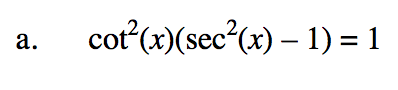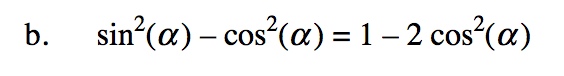### Home > PC3 > Chapter 8 > Lesson 8.3.2 > Problem8-99

8-99.
1. Verify the following trigonometric identities. Homework Help ✎

1. cot2(x)(sec2(x) – 1) = 1

2. sin2(α ) – cos2(α ) = 1 – 2 cos2(α )Substitute a Pythagorean Identity for sec2(x) − 1.Substitute the Fundamental Pythagorean Identity into the left side of the equation for sin2(α).